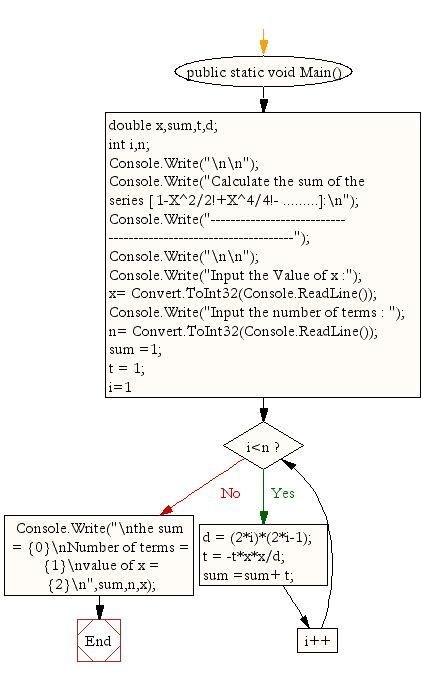﻿ C# - Calculate the sum of the series [ 1-X^2/2!+X^4/4!- ...]# C# Sharp Exercises: Calculate the sum of the series [ 1-X^2/2!+X^4/4!- .........]

## C# Sharp For Loop: Exercise-18 with Solution

Write a program in C# Sharp to find the sum of the series [ 1-X^2/2!+X^4/4!- .........].

Sample Solution:

C# Sharp Code:

``````using System;
public class Exercise18
{
public static void Main()
{
double x,sum,t,d;
int i,n;

Console.Write("\n\n");
Console.Write("Calculate the sum of the series [ 1-X^2/2!+X^4/4!- .........]:\n");
Console.Write("----------------------------------------------------------------");
Console.Write("\n\n");

Console.Write("Input the Value of x :");

Console.Write("Input the number of terms : ");
sum =1; t = 1;
for (i=1;i<n;i++)
{
d = (2*i)*(2*i-1);
t = -t*x*x/d;
sum =sum+ t;
}
Console.Write("\nthe sum = {0}\nNumber of terms = {1}\nvalue of x = {2}\n",sum,n,x);
}
}
```
```

Sample Output:

```Calculate the sum of the series [ 1-X^2/2!+X^4/4!- .........]:
----------------------------------------------------------------
Input the Value of x :5
Input the number of terms : 2
the sum = -11.5
Number of terms = 2
value of x = 5
```

Flowchart:C# Sharp Code Editor:

Contribute your code and comments through Disqus.

What is the difficulty level of this exercise?

Test your Programming skills with w3resource's quiz.

﻿